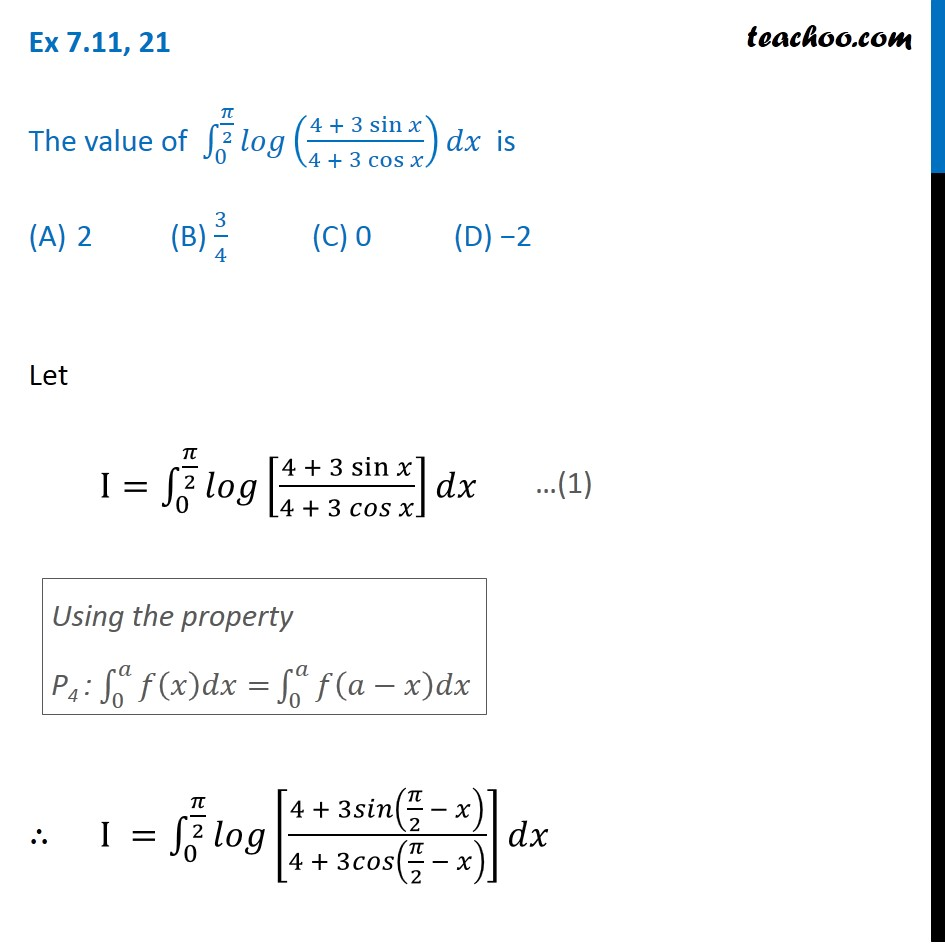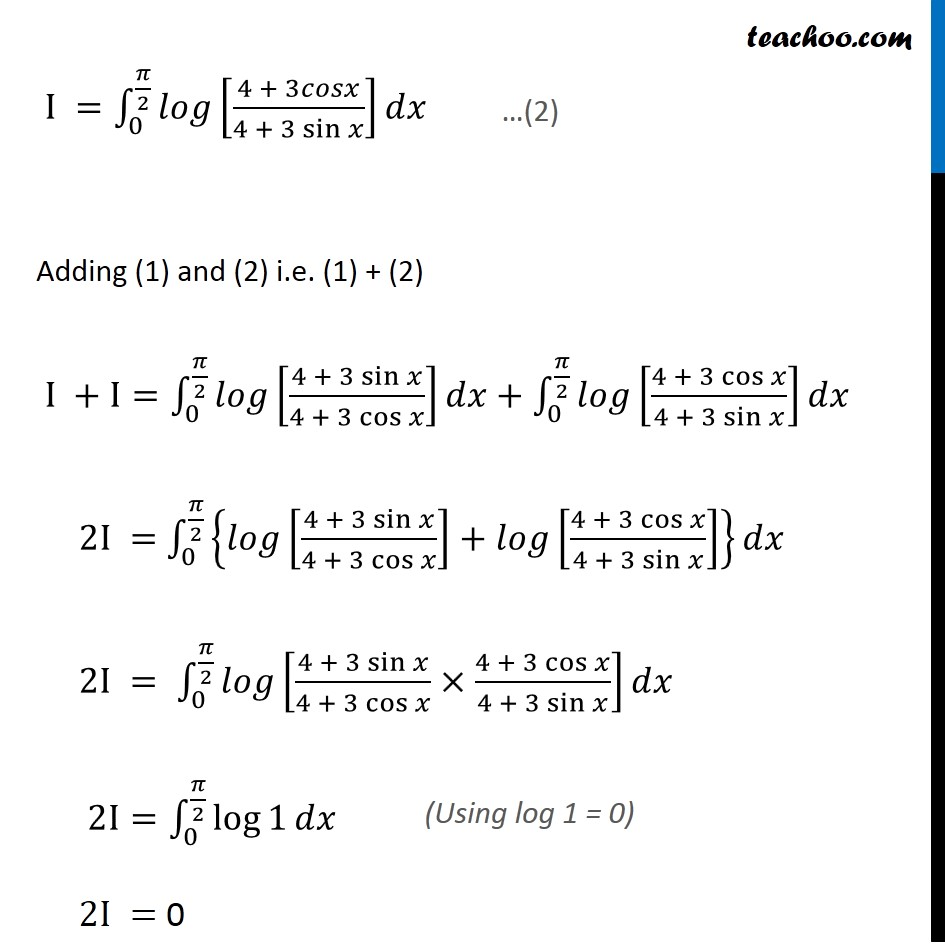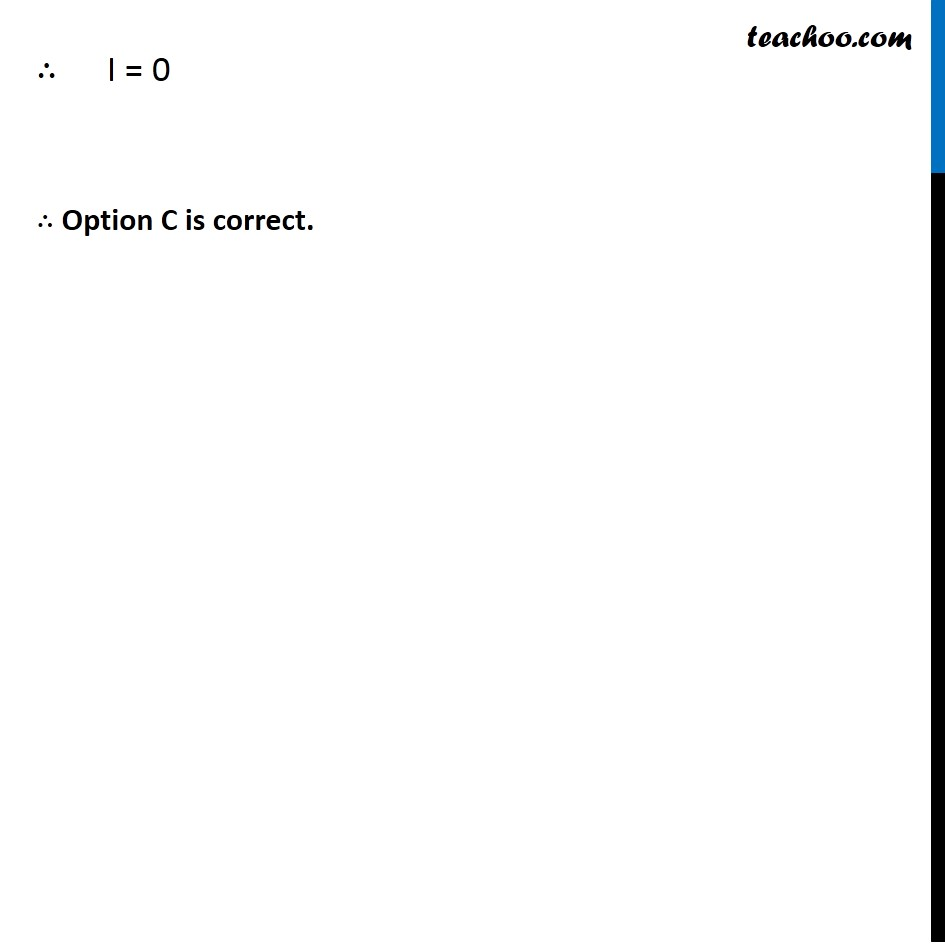Ex 7.10

Chapter 7 Class 12 Integrals
Serial order wiseLearn in your speed, with individual attention - Teachoo Maths 1-on-1 Class

### Transcript

Ex 7.10, 21 The value of ∫_0^(𝜋/2)▒𝑙𝑜𝑔((4 + 3 sin⁡𝑥)/(4 + 3 cos⁡𝑥 ))𝑑𝑥 is 2 (B) 3/4 (C) 0 (D) −2 Let I=∫_0^(𝜋/2)▒𝑙𝑜𝑔[(4 + 3 sin⁡𝑥)/(4 + 3 𝑐𝑜𝑠 𝑥)] 𝑑𝑥 ∴ I =∫_0^(𝜋/2)▒𝑙𝑜𝑔[(4 + 3𝑠𝑖𝑛(𝜋/2 − 𝑥))/(4 + 3𝑐𝑜𝑠(𝜋/2 − 𝑥) )] 𝑑𝑥 Using the property P4 : ∫_0^𝑎▒〖𝑓(𝑥)𝑑𝑥=〗 ∫_0^𝑎▒𝑓(𝑎−𝑥)𝑑𝑥 I =∫_0^(𝜋/2)▒𝑙𝑜𝑔[(4 + 3𝑐𝑜𝑠𝑥)/(4 + 3 sin⁡𝑥 )] 𝑑𝑥 Adding (1) and (2) i.e. (1) + (2) I +I=∫_0^(𝜋/2)▒𝑙𝑜𝑔[(4 + 3 sin⁡𝑥)/(4 + 3 cos⁡𝑥 )] 𝑑𝑥+∫_0^(𝜋/2)▒𝑙𝑜𝑔[(4 + 3 cos⁡𝑥)/(4 + 3 sin⁡𝑥 )] 𝑑𝑥 2I = ∫_0^(𝜋/2)▒{𝑙𝑜𝑔[(4 + 3 sin⁡𝑥)/(4 + 3 cos⁡𝑥 )]+𝑙𝑜𝑔[(4 + 3 cos⁡𝑥)/(4 + 3 sin⁡𝑥 )]}𝑑𝑥" " 2I = ∫_0^(𝜋/2)▒𝑙𝑜𝑔[(4 + 3 sin⁡𝑥)/(4 + 3 cos⁡𝑥 )×(4 + 3 cos⁡𝑥)/(4 + 3 sin⁡𝑥 )]𝑑𝑥" " 2I=∫_0^(𝜋/2)▒〖log⁡1 𝑑𝑥〗 2I = 0 ∴ I = 0 ∴ Option C is correct.